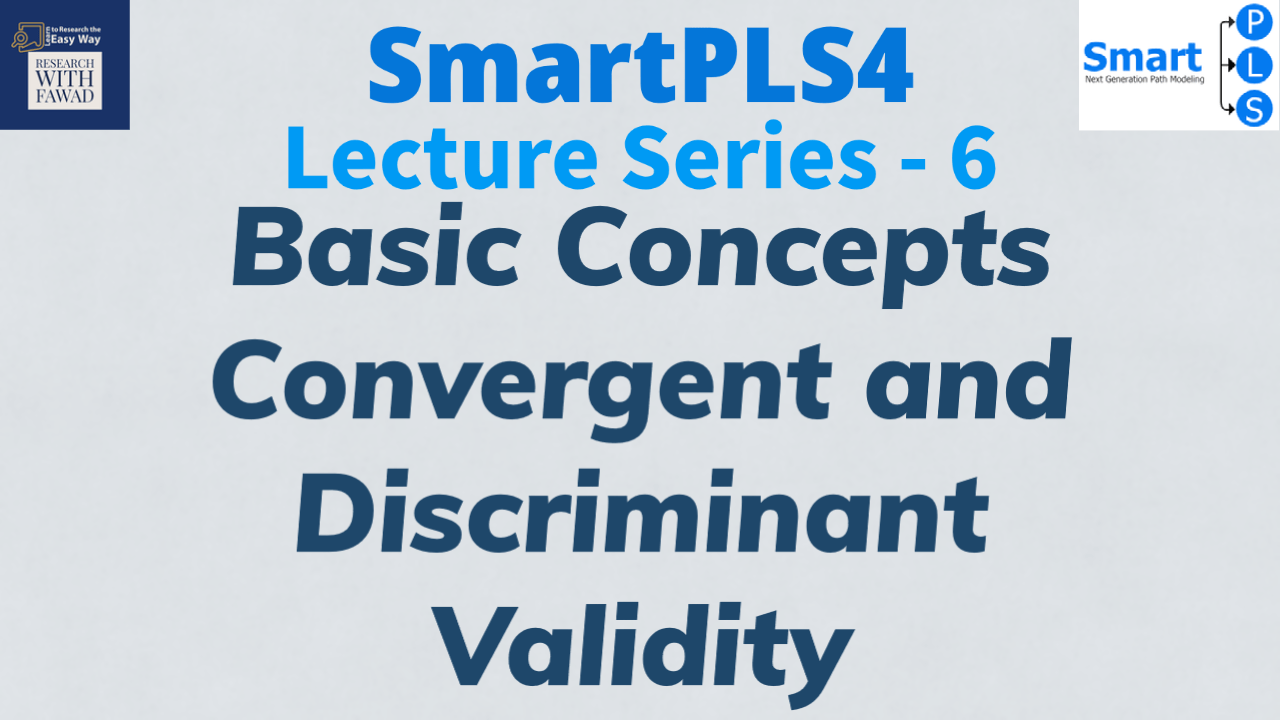# Basic Concepts in SEM: Convergent and Discriminant Validity## Basic Structural Equation Modelling Concepts

In Structural Equation Modelling, a model is based on assessment of Measurement and Structural Model. First, measurement measurement models are assessed on the grounds of indicator reliability, internal consistency reliability, convergent validity, and discriminant validity.

Finally, Structural Model are assessed to substantiate the proposed hypotheses. In this section, i briefly discuss the concept of convergent and discriminant validity. The last session discussed the basic SEM concepts including Loadings and Reliability.

### What is Convergent Validity?

Validity assessment focuses on each measure’s convergent validity using the Average Variance Extracted (AVE). Convergent validity is the extent to which the construct converges in order to explain the variance of its indicators. The metric used for evaluating a construct’s convergent validity is the average variance extracted (AVE) for all indicators on each construct.

The AVE is defined as the grand mean value of the squared loadings of the indicators associated with the construct (i.e., the sum of the squared loadings divided by the number of indicators). Therefore, the AVE is equivalent to the communality of a construct.Convergent Validity is established if the AVE value is greater than or equal to 0.50.

### What is Discriminant Validity?

This metric measures the extent to which a construct is empirically distinct from other constructs in the structural model. The most common metrics used to establish discriminant validity include Fornell & Larcker (1981) criterion and Heterotrait-Monotrait Ratio.

### Reference

Hair Jr, J. F., Hult, G. T. M., Ringle, C. M., Sarstedt, M., Danks, N. P., & Ray, S. (2021). Partial least squares structural equation modeling (PLS-SEM) using R: A workbook.